# 循环嵌套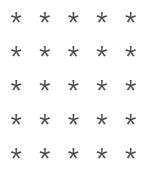print('* * * * *')
print('* * * * *')
print('* * * * *')
print('* * * * *')
print('* * * * *')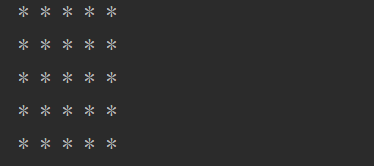i=0
while i<5 :
print('* * * * *')
i+=1

i=0
while i<5 :
j=0
while j<5:
print('* ')
j+=1
i+=1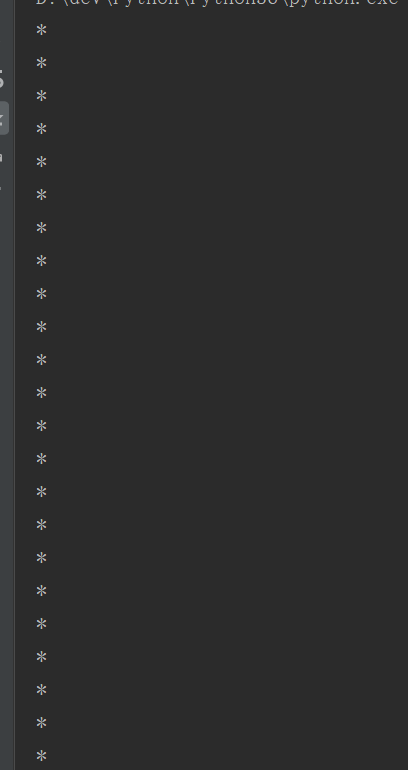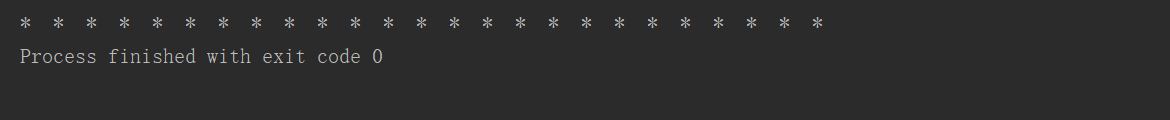i=0
while i<5 :
j=0
while j<5:
print('* ',end=' ')
j+=1
print()
i+=1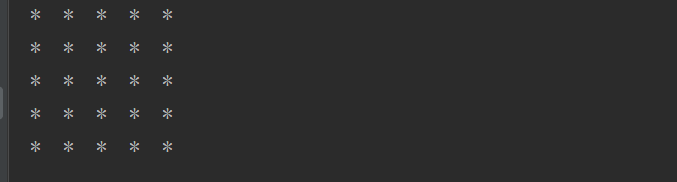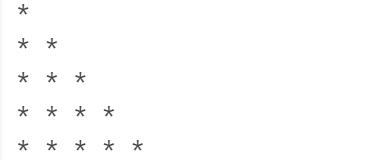i=0
while i<5 :
j=0
while j<i+1:
print('* ',end=' ')
j+=1
print()
i+=1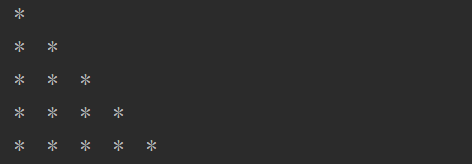1*1=1
1*2=2 2*2=4
1*3=3 2*3=6 3*3=9
...9*9=81

## 视频学习：阿里云大学之Python进阶必看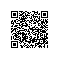Python学习资料大全